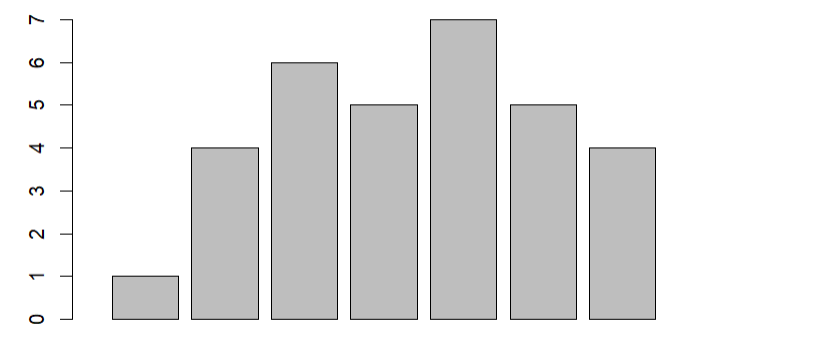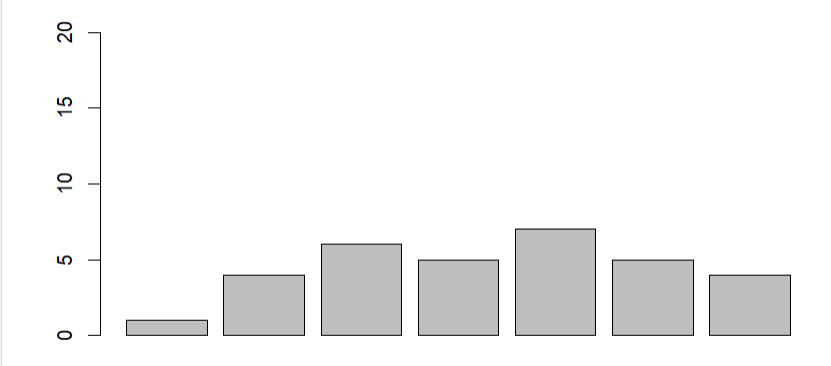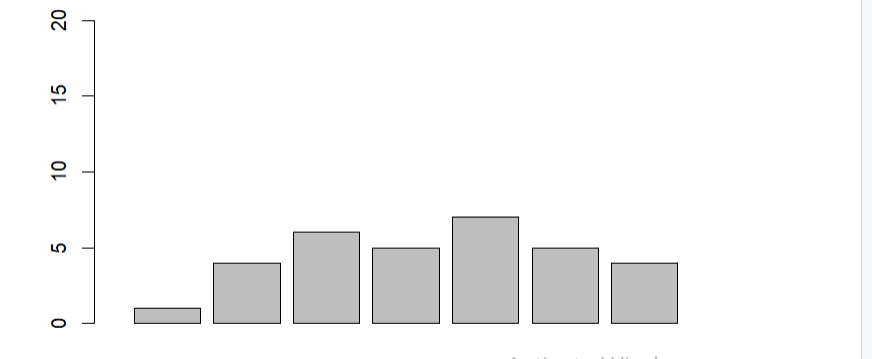Open in App
Not now

# How to Use xlim() and ylim() in R?

• Last Updated : 28 Nov, 2021

In this article, we will be looking at the different ways to use the xlim() and the ylim() functions in the R programming language.

## Method 1: Using xlim() to Set X-Axis Limits

The xlim() function with the provided parameters as the range of the x-axis in vectors is used to set the x-axis without dropping any data of the given plot or an object accordingly.

Syntax:

`xlim(...)`

Parameters:

• …: if numeric, will create a continuous scale, if factor or character, will create a discrete scale.

Example:

In this example, we will be using the xlim() function to change the x-axis of the given bar plot in the R programming language.

## R

 `# Datapoint for the barplot``gfg<-``c``(1,4,6,5,7,5,4)``   ` `# Creating bar plot and setting ``# the x-axis limits``barplot``(gfg,xlim=``c``(0,10))`

Output:## Method 2: Use ylim() to Set Y-Axis Limits

The xlim() function with the provided parameters as the range of the y-axis in vectors is used to set the y-axis without dropping any data of the given plot or an object accordingly.

Syntax:

`xlim(...)`

Parameters:

• …: if numeric, will create a continuous scale, if factor or character, will create a discrete scale.

Example:

In this example, we will be using the ylim() function to change the y-axis of the given bar plot (with the same data as in the previous example)in the R programming language.

## R

 `# Datapoint for the barplot``gfg<-``c``(1,4,6,5,7,5,4)`` ` `# Creating bar plot and setting ``# the y-axis limits``barplot``(gfg,ylim=``c``(0,20))`

Output:## Method 3 : Use xlim() & ylim() to Set Axis Limits

Here, we will be using both xlim() and the ylim() function together to set the axis limits accordingly of both the x-axis and y-axis together in the same plot to get a clear idea of the outcomes, refer to the below example.

Example:

In this example, we will be using the xlim() and the ylim() function both together in the same barplot with the same data as the previous example and set the axis limits accordingly in the R programming language.

## R

 `# Datapoint for the barplot``gfg<-``c``(1,4,6,5,7,5,4)`` ` `# Creating bar plot and setting the``# x-axis and y-axis limits``barplot``(gfg,xlim=``c``(0,10),ylim=``c``(0,20))`

Output:My Personal Notes arrow_drop_up